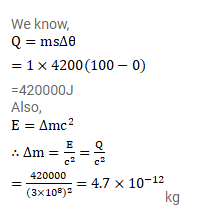# Find the increase in mass when 1kg of water is heated from 0°c to 1000c.

Question:

Find the increase in mass when $1 \mathrm{~kg}$ of water is heated from $0^{\circ} \mathrm{C}$ to $100^{\circ} \mathrm{C}$. Specific heat capacity of water=4200 $\mathrm{J} / \mathrm{kg}-\mathrm{K}$.

Solution: